Скачать презентацию UNIVERSITY OF MASSACHUSETTS Dept of Electrical Computer

7490b09b772373c62e3422f92d2ea9c6.ppt

• Количество слайдов: 31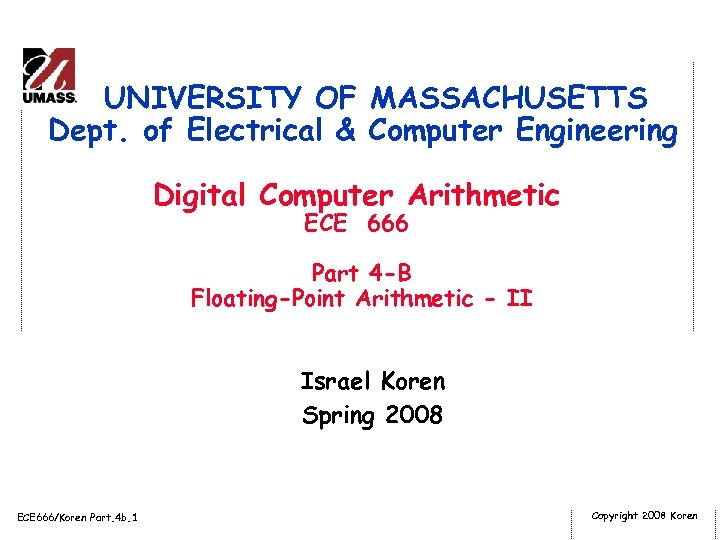UNIVERSITY OF MASSACHUSETTS Dept. of Electrical & Computer Engineering Digital Computer Arithmetic ECE 666 Part 4 -B Floating-Point Arithmetic - II Israel Koren Spring 2008 ECE 666/Koren Part. 4 b. 1 Copyright 2008 Koren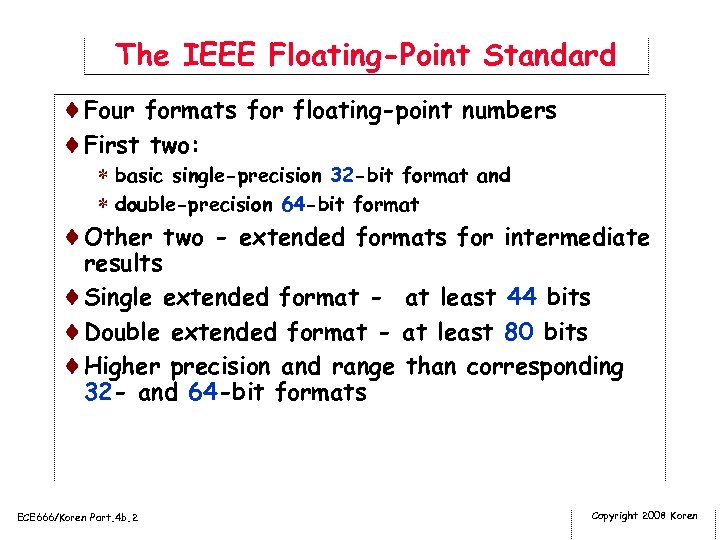The IEEE Floating-Point Standard ¨Four formats for floating-point numbers ¨First two: * basic single-precision 32 -bit format and * double-precision 64 -bit format ¨Other two - extended formats for intermediate results ¨Single extended format - at least 44 bits ¨Double extended format - at least 80 bits ¨Higher precision and range than corresponding 32 - and 64 -bit formats ECE 666/Koren Part. 4 b. 2 Copyright 2008 Koren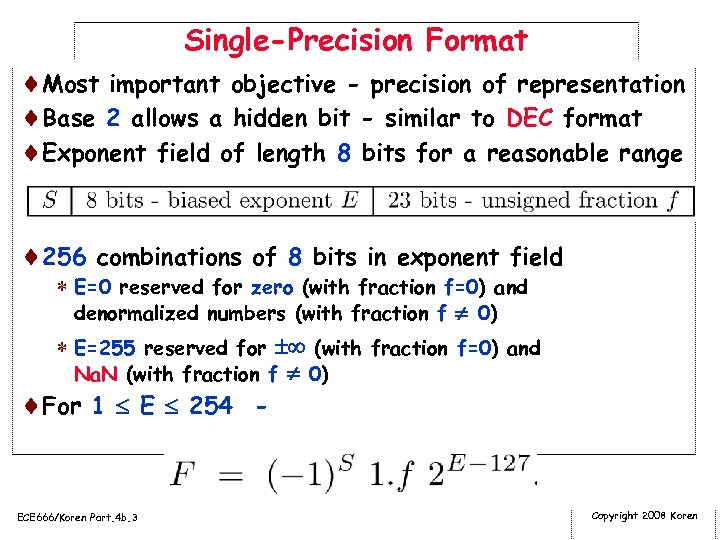Single-Precision Format ¨Most important objective - precision of representation ¨Base 2 allows a hidden bit - similar to DEC format ¨Exponent field of length 8 bits for a reasonable range ¨ 256 combinations of 8 bits in exponent field * E=0 reserved for zero (with fraction f=0) and denormalized numbers (with fraction f 0) * E=255 reserved for (with fraction f=0) and Na. N (with fraction f 0) ¨For 1 E 254 - ECE 666/Koren Part. 4 b. 3 Copyright 2008 Koren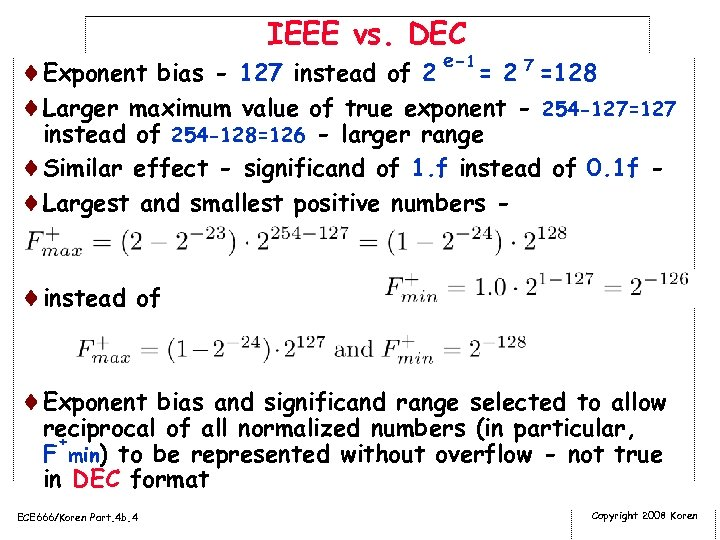IEEE vs. DEC e-1 ¨Exponent bias - 127 instead of 2 = 2 7 =128 ¨Larger maximum value of true exponent - 254 -127=127 instead of 254 -128=126 - larger range ¨Similar effect - significand of 1. f instead of 0. 1 f ¨Largest and smallest positive numbers - ¨instead of ¨Exponent bias and significand range selected to allow reciprocal of all normalized numbers (in particular, + F min) to be represented without overflow - not true in DEC format ECE 666/Koren Part. 4 b. 4 Copyright 2008 Koren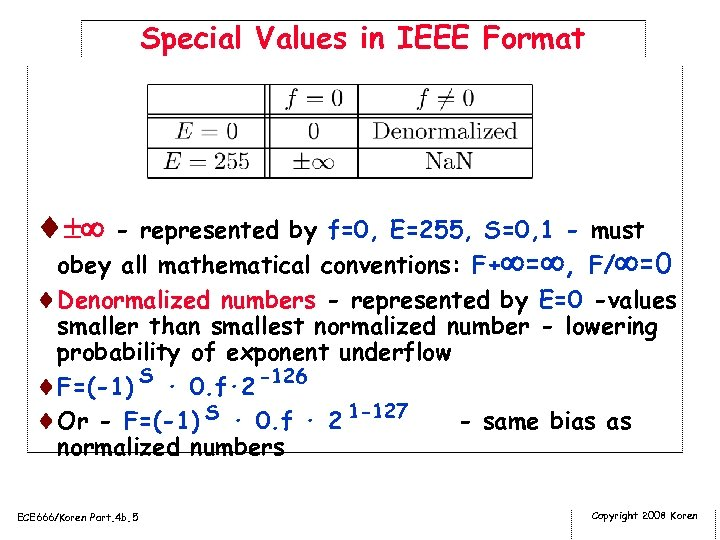Special Values in IEEE Format ¨ - represented by f=0, E=255, S=0, 1 - must obey all mathematical conventions: F+ = , F/ =0 ¨Denormalized numbers - represented by E=0 -values smaller than smallest normalized number - lowering probability of exponent underflow ¨F=(-1) S · 0. f· 2 -126 ¨Or - F=(-1) S · 0. f · 2 1 -127 - same bias as normalized numbers ECE 666/Koren Part. 4 b. 5 Copyright 2008 Koren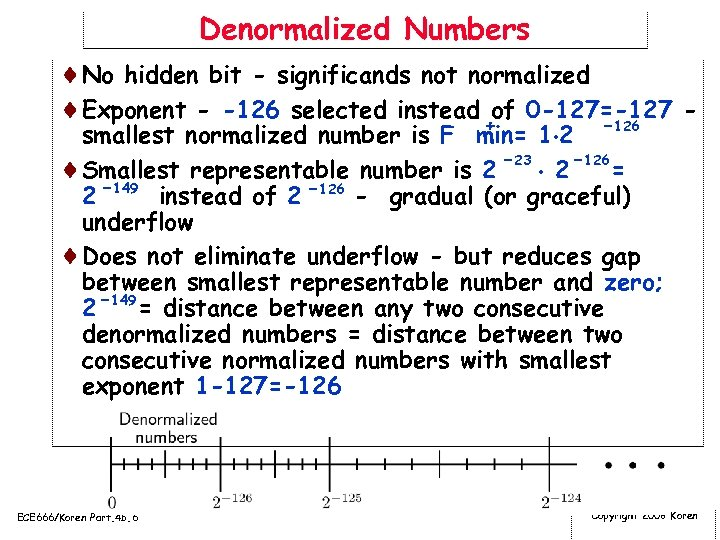Denormalized Numbers ¨No hidden bit - significands not normalized ¨Exponent - -126 selected instead of 0 -127=-127 -126 + smallest normalized number is F min= 1 2 ¨Smallest representable number is 2 -23 2 -126 = 2 -149 instead of 2 -126 - gradual (or graceful) underflow ¨Does not eliminate underflow - but reduces gap between smallest representable number and zero; 2 -149 = distance between any two consecutive denormalized numbers = distance between two consecutive normalized numbers with smallest exponent 1 -127=-126 ECE 666/Koren Part. 4 b. 6 Copyright 2008 Koren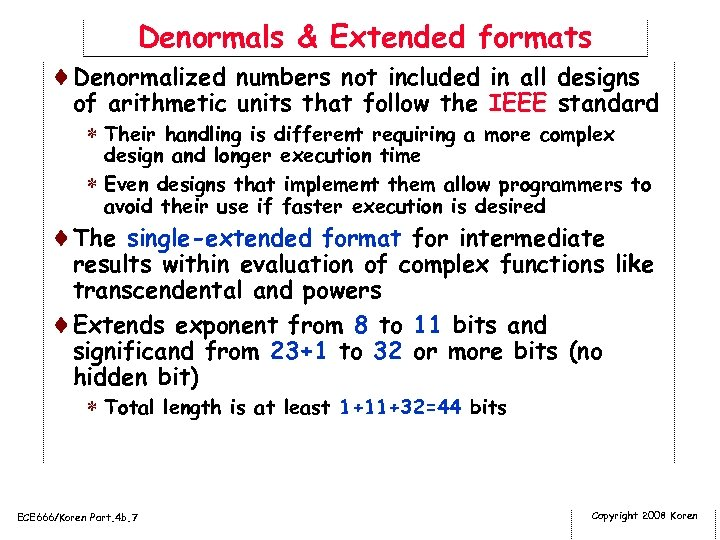Denormals & Extended formats ¨Denormalized numbers not included in all designs of arithmetic units that follow the IEEE standard * Their handling is different requiring a more complex design and longer execution time * Even designs that implement them allow programmers to avoid their use if faster execution is desired ¨The single-extended format for intermediate results within evaluation of complex functions like transcendental and powers ¨Extends exponent from 8 to 11 bits and significand from 23+1 to 32 or more bits (no hidden bit) * Total length is at least 1+11+32=44 bits ECE 666/Koren Part. 4 b. 7 Copyright 2008 Koren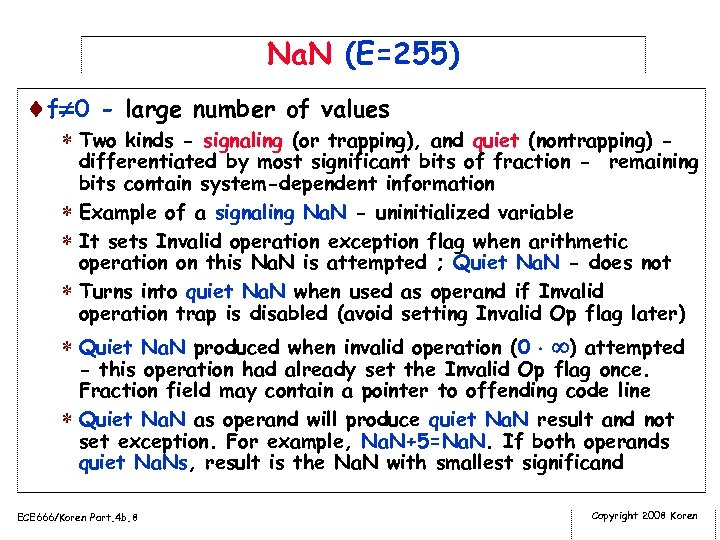Na. N (E=255) ¨f 0 - large number of values * Two kinds - signaling (or trapping), and quiet (nontrapping) - differentiated by most significant bits of fraction - remaining bits contain system-dependent information * Example of a signaling Na. N - uninitialized variable * It sets Invalid operation exception flag when arithmetic operation on this Na. N is attempted ; Quiet Na. N - does not * Turns into quiet Na. N when used as operand if Invalid operation trap is disabled (avoid setting Invalid Op flag later) * Quiet Na. N produced when invalid operation (0 ) attempted - this operation had already set the Invalid Op flag once. Fraction field may contain a pointer to offending code line * Quiet Na. N as operand will produce quiet Na. N result and not set exception. For example, Na. N+5=Na. N. If both operands quiet Na. Ns, result is the Na. N with smallest significand ECE 666/Koren Part. 4 b. 8 Copyright 2008 Koren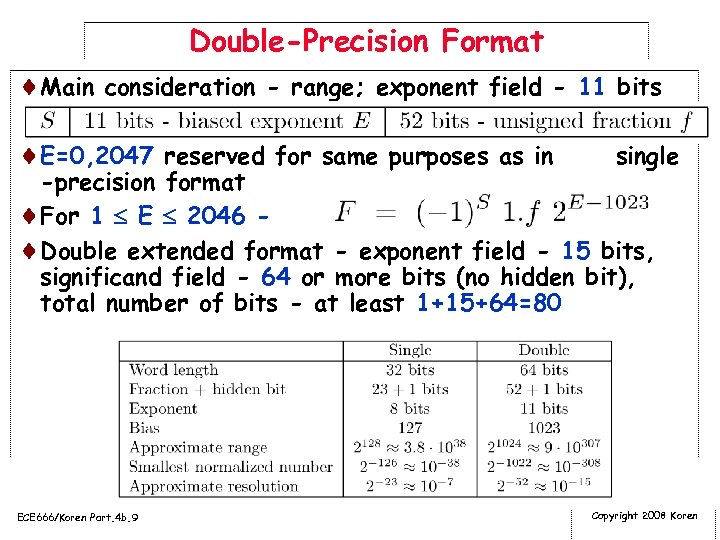Double-Precision Format ¨Main consideration - range; exponent field - 11 bits ¨E=0, 2047 reserved for same purposes as in single -precision format ¨For 1 E 2046 ¨Double extended format - exponent field - 15 bits, significand field - 64 or more bits (no hidden bit), total number of bits - at least 1+15+64=80 ECE 666/Koren Part. 4 b. 9 Copyright 2008 Koren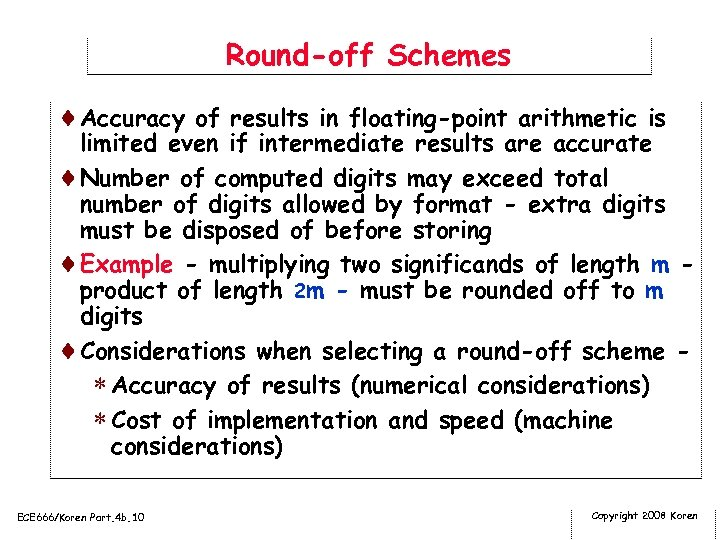Round-off Schemes ¨Accuracy of results in floating-point arithmetic is limited even if intermediate results are accurate ¨Number of computed digits may exceed total number of digits allowed by format - extra digits must be disposed of before storing ¨Example - multiplying two significands of length m product of length 2 m - must be rounded off to m digits ¨Considerations when selecting a round-off scheme * Accuracy of results (numerical considerations) * Cost of implementation and speed (machine considerations) ECE 666/Koren Part. 4 b. 10 Copyright 2008 Koren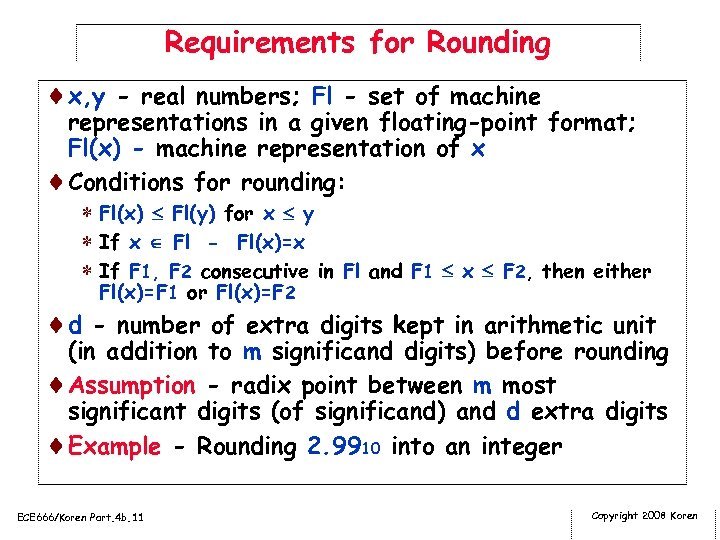Requirements for Rounding ¨x, y - real numbers; Fl - set of machine representations in a given floating-point format; Fl(x) - machine representation of x ¨Conditions for rounding: * Fl(x) Fl(y) for x y * If x Fl - Fl(x)=x * If F 1, F 2 consecutive in Fl and F 1 x F 2, then either Fl(x)=F 1 or Fl(x)=F 2 ¨d - number of extra digits kept in arithmetic unit (in addition to m significand digits) before rounding ¨Assumption - radix point between m most significant digits (of significand) and d extra digits ¨Example - Rounding 2. 9910 into an integer ECE 666/Koren Part. 4 b. 11 Copyright 2008 Koren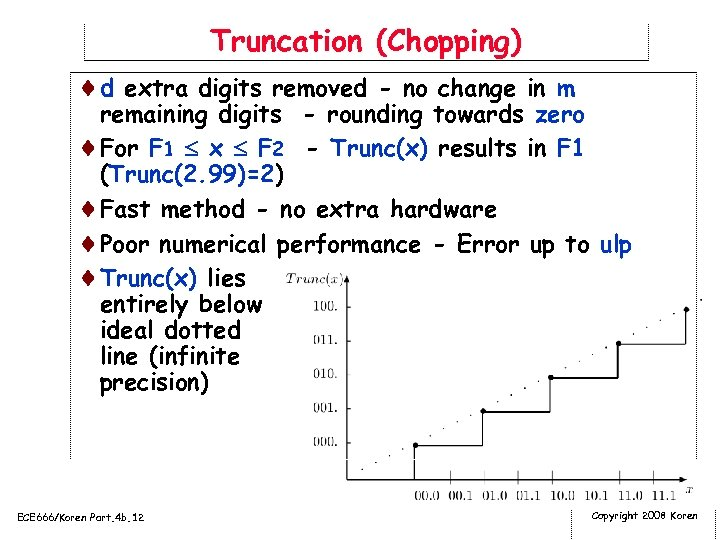Truncation (Chopping) ¨d extra digits removed - no change in m remaining digits - rounding towards zero ¨For F 1 x F 2 - Trunc(x) results in F 1 (Trunc(2. 99)=2) ¨Fast method - no extra hardware ¨Poor numerical performance - Error up to ulp ¨Trunc(x) lies entirely below ideal dotted line (infinite precision) ECE 666/Koren Part. 4 b. 12 Copyright 2008 Koren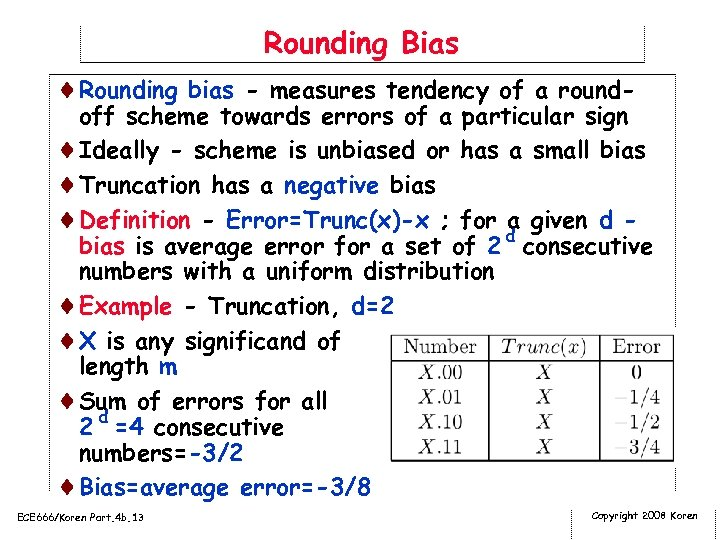Rounding Bias ¨Rounding bias - measures tendency of a round- off scheme towards errors of a particular sign ¨Ideally - scheme is unbiased or has a small bias ¨Truncation has a negative bias ¨Definition - Error=Trunc(x)-x ; for a given d bias is average error for a set of 2 d consecutive numbers with a uniform distribution ¨Example - Truncation, d=2 ¨X is any significand of length m ¨Sum of errors for all 2 d =4 consecutive numbers=-3/2 ¨Bias=average error=-3/8 ECE 666/Koren Part. 4 b. 13 Copyright 2008 Koren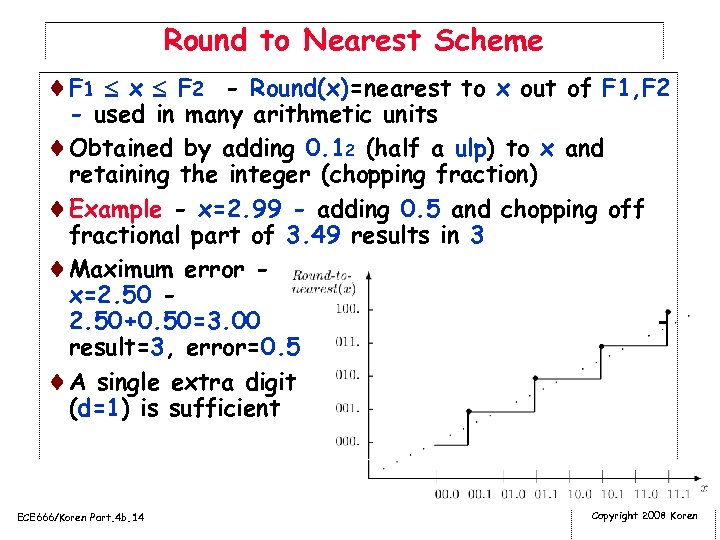Round to Nearest Scheme ¨F 1 x F 2 - Round(x)=nearest to x out of F 1, F 2 - used in many arithmetic units ¨Obtained by adding 0. 12 (half a ulp) to x and retaining the integer (chopping fraction) ¨Example - x=2. 99 - adding 0. 5 and chopping off fractional part of 3. 49 results in 3 ¨Maximum error x=2. 50+0. 50=3. 00 result=3, error=0. 5 ¨A single extra digit (d=1) is sufficient ECE 666/Koren Part. 4 b. 14 Copyright 2008 Koren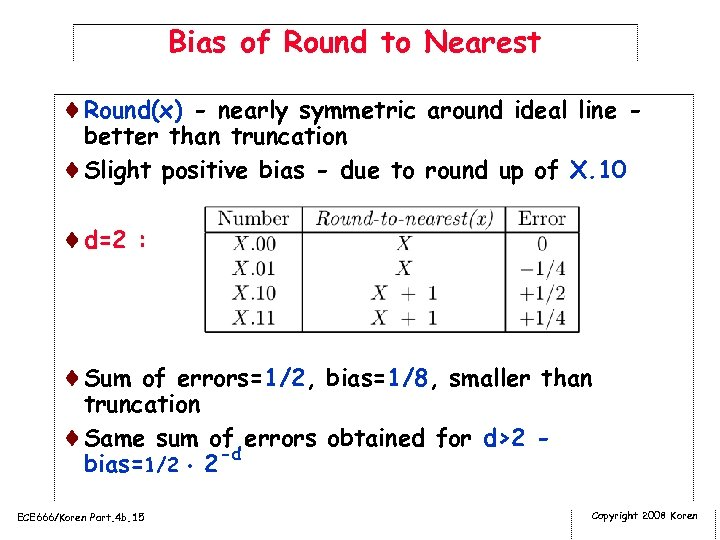Bias of Round to Nearest ¨Round(x) - nearly symmetric around ideal line better than truncation ¨Slight positive bias - due to round up of X. 10 ¨d=2 : ¨Sum of errors=1/2, bias=1/8, smaller than truncation ¨Same sum of errors obtained for d>2 bias=1/2 2 -d ECE 666/Koren Part. 4 b. 15 Copyright 2008 Koren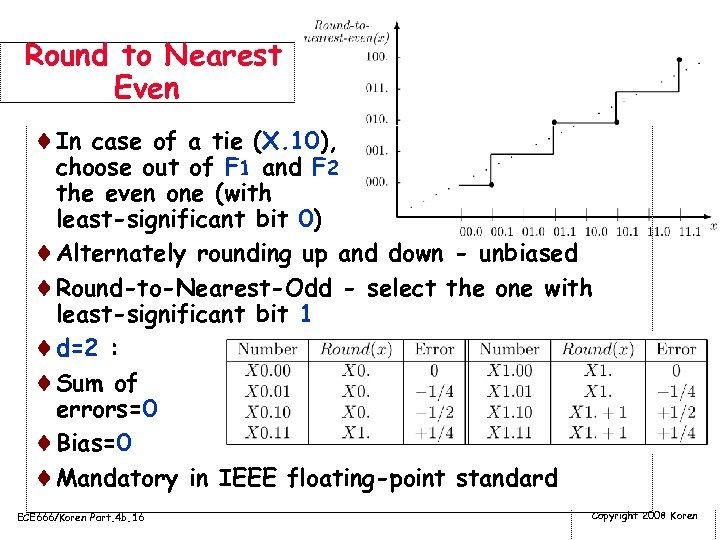Round to Nearest Even ¨In case of a tie (X. 10), choose out of F 1 and F 2 the even one (with least-significant bit 0) ¨Alternately rounding up and down - unbiased ¨Round-to-Nearest-Odd - select the one with least-significant bit 1 ¨d=2 : ¨Sum of errors=0 ¨Bias=0 ¨Mandatory in IEEE floating-point standard ECE 666/Koren Part. 4 b. 16 Copyright 2008 Koren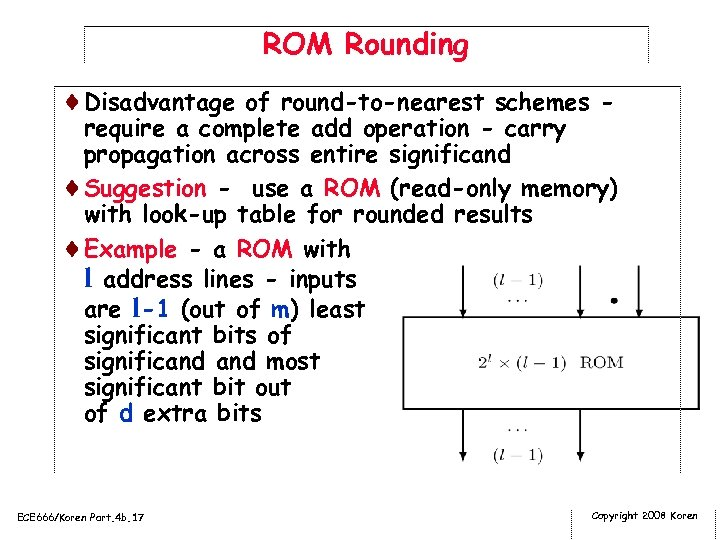ROM Rounding ¨Disadvantage of round-to-nearest schemes - require a complete add operation - carry propagation across entire significand ¨Suggestion - use a ROM (read-only memory) with look-up table for rounded results ¨Example - a ROM with l address lines - inputs are l-1 (out of m) least significant bits of significand most significant bit out of d extra bits ECE 666/Koren Part. 4 b. 17 Copyright 2008 Koren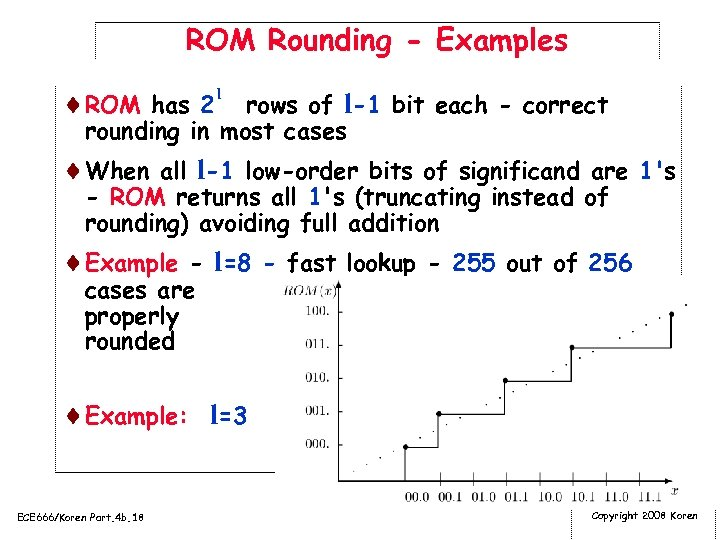ROM Rounding - Examples ¨ROM has 2 l rows of l-1 bit each - correct rounding in most cases ¨When all l-1 low-order bits of significand are 1's - ROM returns all 1's (truncating instead of rounding) avoiding full addition ¨Example - l=8 - fast lookup - 255 out of 256 cases are properly rounded ¨Example: l=3 ECE 666/Koren Part. 4 b. 18 Copyright 2008 Koren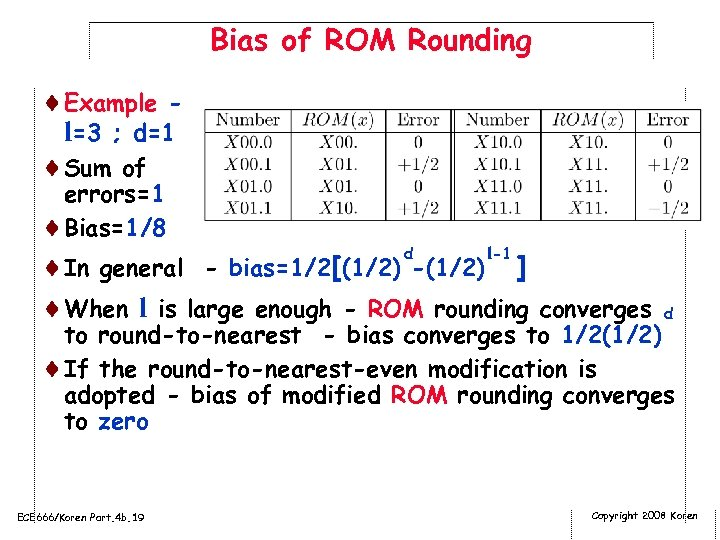Bias of ROM Rounding ¨Example l=3 ; d=1 ¨Sum of errors=1 ¨Bias=1/8 d l-1 ¨In general - bias=1/2[(1/2) -(1/2) ] ¨When l is large enough - ROM rounding converges d to round-to-nearest - bias converges to 1/2(1/2) ¨If the round-to-nearest-even modification is adopted - bias of modified ROM rounding converges to zero ECE 666/Koren Part. 4 b. 19 Copyright 2008 Koren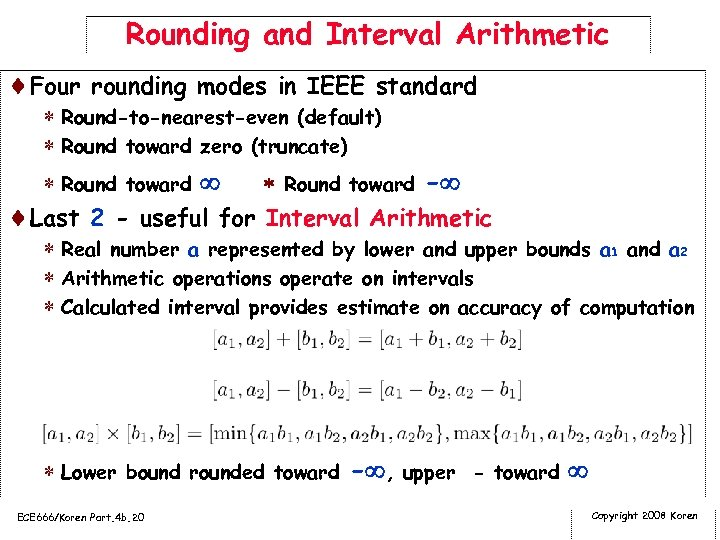Rounding and Interval Arithmetic ¨Four rounding modes in IEEE standard * Round-to-nearest-even (default) * Round toward zero (truncate) * Round toward - ¨Last 2 - useful for Interval Arithmetic * Real number a represented by lower and upper bounds a 1 and a 2 * Arithmetic operations operate on intervals * Calculated interval provides estimate on accuracy of computation * Lower bound rounded toward - , upper - toward ECE 666/Koren Part. 4 b. 20 Copyright 2008 Koren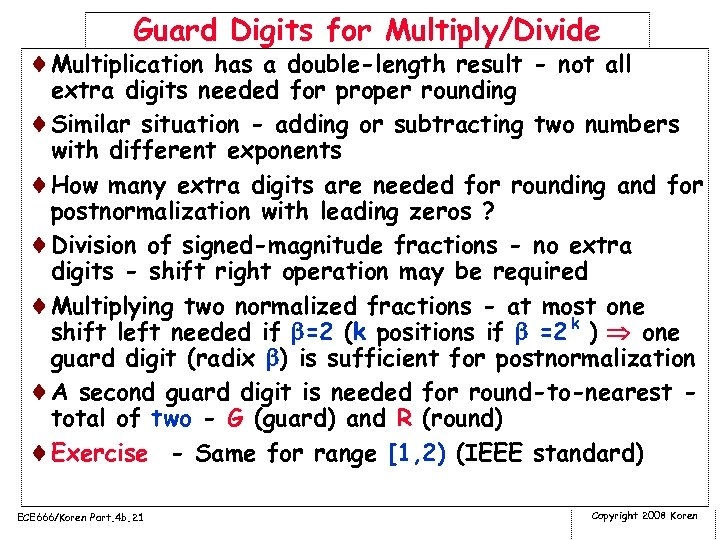Guard Digits for Multiply/Divide ¨Multiplication has a double-length result - not all extra digits needed for proper rounding ¨Similar situation - adding or subtracting two numbers with different exponents ¨How many extra digits are needed for rounding and for postnormalization with leading zeros ? ¨Division of signed-magnitude fractions - no extra digits - shift right operation may be required ¨Multiplying two normalized fractions - at most one shift left needed if =2 (k positions if =2 k ) one guard digit (radix ) is sufficient for postnormalization ¨A second guard digit is needed for round-to-nearest total of two - G (guard) and R (round) ¨Exercise - Same for range [1, 2) (IEEE standard) ECE 666/Koren Part. 4 b. 21 Copyright 2008 Koren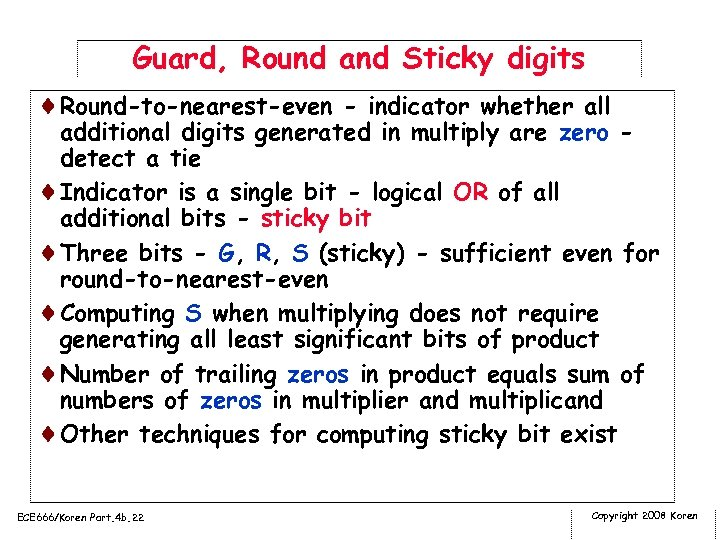Guard, Round and Sticky digits ¨Round-to-nearest-even - indicator whether all additional digits generated in multiply are zero detect a tie ¨Indicator is a single bit - logical OR of all additional bits - sticky bit ¨Three bits - G, R, S (sticky) - sufficient even for round-to-nearest-even ¨Computing S when multiplying does not require generating all least significant bits of product ¨Number of trailing zeros in product equals sum of numbers of zeros in multiplier and multiplicand ¨Other techniques for computing sticky bit exist ECE 666/Koren Part. 4 b. 22 Copyright 2008 Koren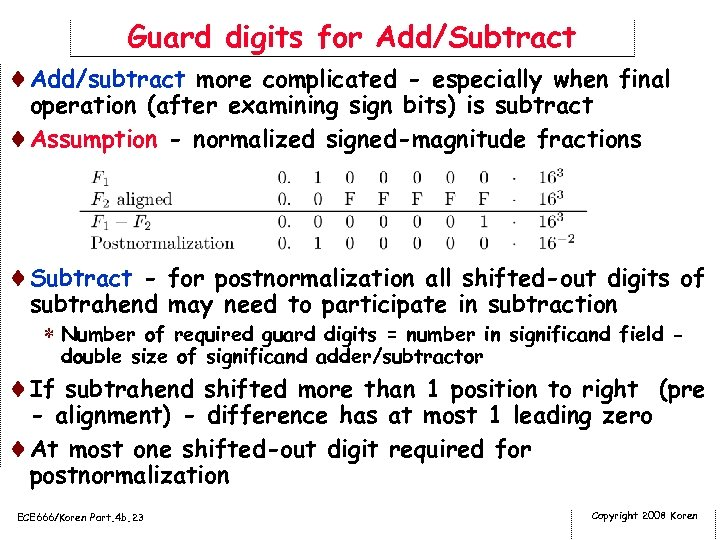Guard digits for Add/Subtract ¨Add/subtract more complicated - especially when final operation (after examining sign bits) is subtract ¨Assumption - normalized signed-magnitude fractions ¨Subtract - for postnormalization all shifted-out digits of subtrahend may need to participate in subtraction * Number of required guard digits = number in significand field double size of significand adder/subtractor ¨If subtrahend shifted more than 1 position to right (pre - alignment) - difference has at most 1 leading zero ¨At most one shifted-out digit required for postnormalization ECE 666/Koren Part. 4 b. 23 Copyright 2008 Koren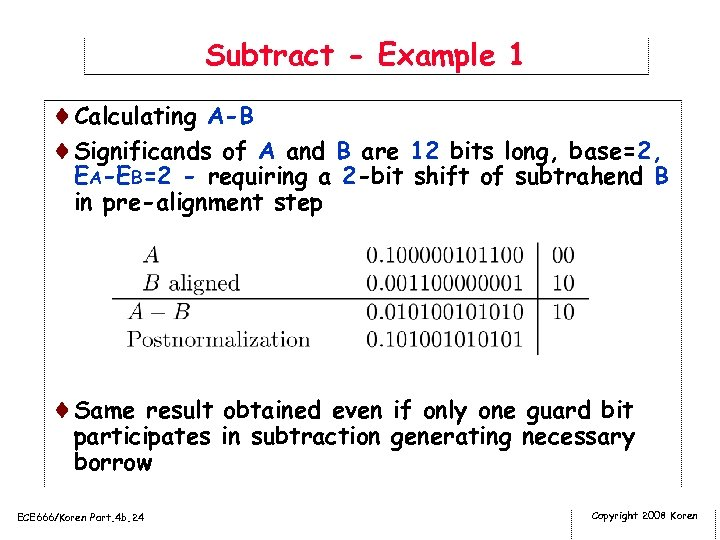Subtract - Example 1 ¨Calculating A-B ¨Significands of A and B are 12 bits long, base=2, EA-EB=2 - requiring a 2 -bit shift of subtrahend B in pre-alignment step ¨Same result obtained even if only one guard bit participates in subtraction generating necessary borrow ECE 666/Koren Part. 4 b. 24 Copyright 2008 Koren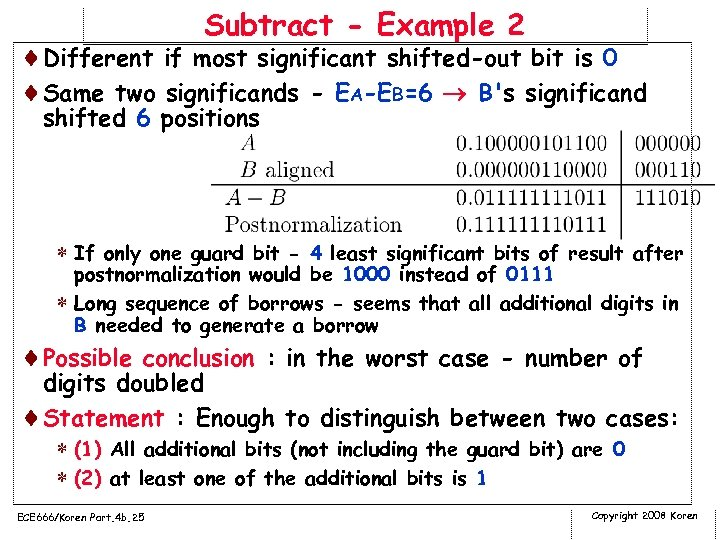Subtract - Example 2 ¨Different if most significant shifted-out bit is 0 ¨Same two significands - EA-EB=6 B's significand shifted 6 positions * If only one guard bit - 4 least significant bits of result after postnormalization would be 1000 instead of 0111 * Long sequence of borrows - seems that all additional digits in B needed to generate a borrow ¨Possible conclusion : in the worst case - number of digits doubled ¨Statement : Enough to distinguish between two cases: * (1) All additional bits (not including the guard bit) are 0 * (2) at least one of the additional bits is 1 ECE 666/Koren Part. 4 b. 25 Copyright 2008 Koren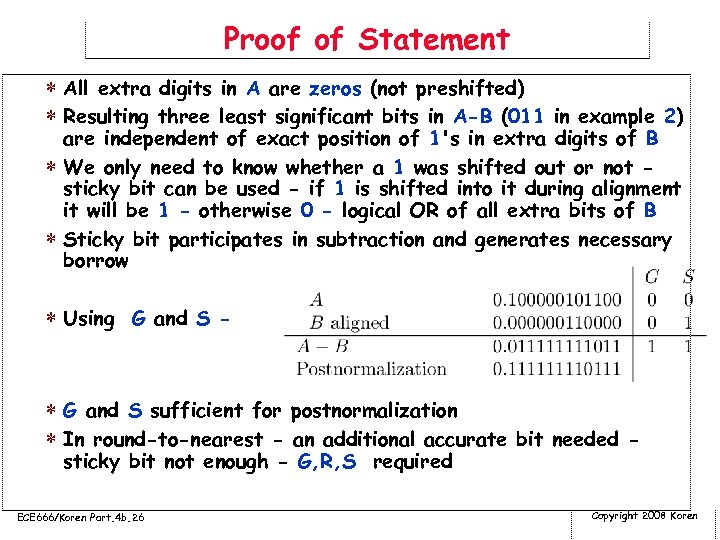Proof of Statement * All extra digits in A are zeros (not preshifted) * Resulting three least significant bits in A-B (011 in example 2) are independent of exact position of 1's in extra digits of B * We only need to know whether a 1 was shifted out or not sticky bit can be used - if 1 is shifted into it during alignment it will be 1 - otherwise 0 - logical OR of all extra bits of B * Sticky bit participates in subtraction and generates necessary borrow * Using G and S - * G and S sufficient for postnormalization * In round-to-nearest - an additional accurate bit needed sticky bit not enough - G, R, S required ECE 666/Koren Part. 4 b. 26 Copyright 2008 Koren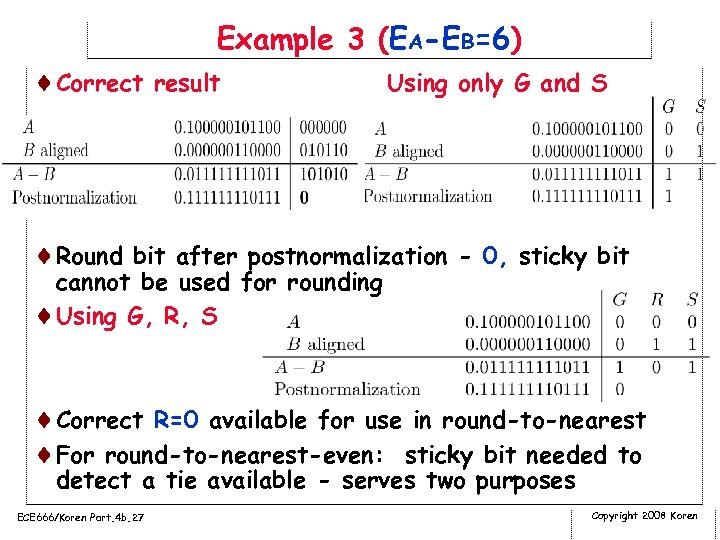Example 3 (EA-EB=6) ¨Correct result Using only G and S ¨Round bit after postnormalization - 0, sticky bit cannot be used for rounding ¨Using G, R, S ¨Correct R=0 available for use in round-to-nearest ¨For round-to-nearest-even: sticky bit needed to detect a tie available - serves two purposes ECE 666/Koren Part. 4 b. 27 Copyright 2008 Koren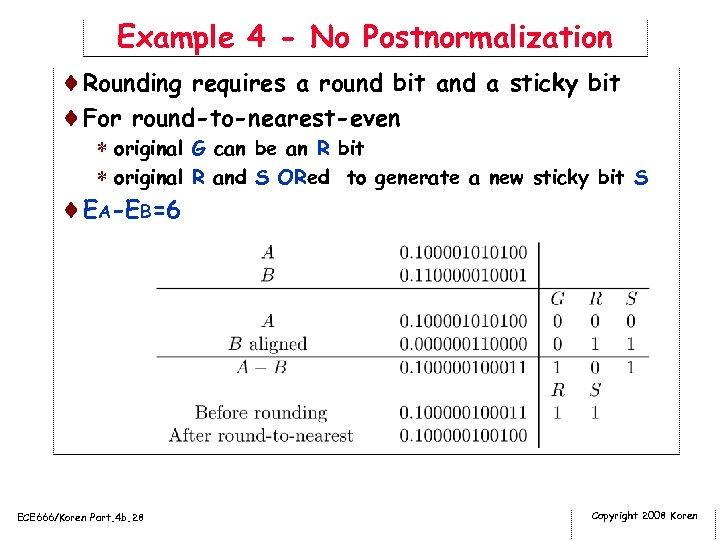Example 4 - No Postnormalization ¨Rounding requires a round bit and a sticky bit ¨For round-to-nearest-even * original G can be an R bit * original R and S ORed to generate a new sticky bit S ¨EA-EB=6 ECE 666/Koren Part. 4 b. 28 Copyright 2008 Koren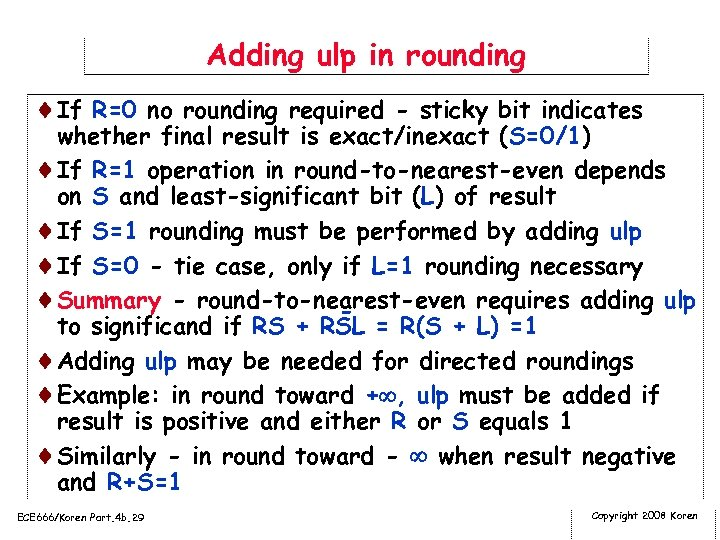Adding ulp in rounding ¨If R=0 no rounding required - sticky bit indicates whether final result is exact/inexact (S=0/1) ¨If R=1 operation in round-to-nearest-even depends on S and least-significant bit (L) of result ¨If S=1 rounding must be performed by adding ulp ¨If S=0 - tie case, only if L=1 rounding necessary ¨Summary - round-to-nearest-even requires adding ulp to significand if RS + RSL = R(S + L) =1 ¨Adding ulp may be needed for directed roundings ¨Example: in round toward + , ulp must be added if result is positive and either R or S equals 1 ¨Similarly - in round toward - when result negative and R+S=1 ECE 666/Koren Part. 4 b. 29 Copyright 2008 Koren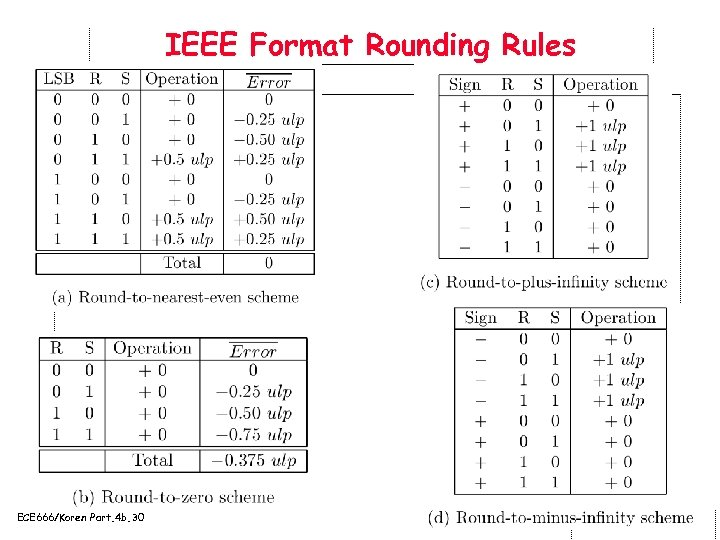IEEE Format Rounding Rules ¨a ECE 666/Koren Part. 4 b. 30 Copyright 2008 Koren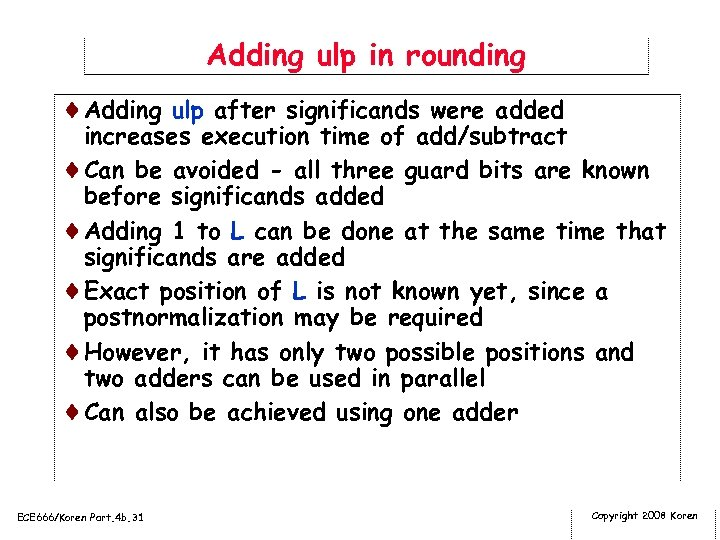Adding ulp in rounding ¨Adding ulp after significands were added increases execution time of add/subtract ¨Can be avoided - all three guard bits are known before significands added ¨Adding 1 to L can be done at the same time that significands are added ¨Exact position of L is not known yet, since a postnormalization may be required ¨However, it has only two possible positions and two adders can be used in parallel ¨Can also be achieved using one adder ECE 666/Koren Part. 4 b. 31 Copyright 2008 Koren